# Linear Equations Linear equations are functions which have

• Slides: 11Linear Equations Linear equations are functions which have two variables. They have an independent and dependent variable.Independent and Dependent Variables Independent variables are variables that you put into the equations Dependent Variables Dependent variables are variables that you solve for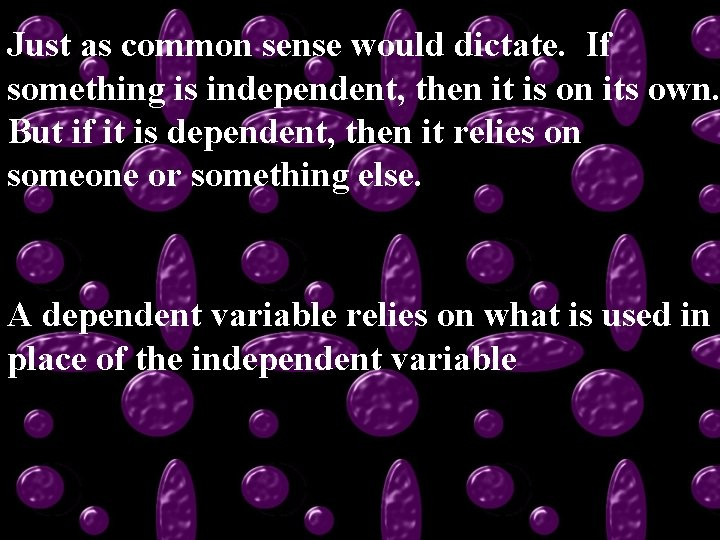Just as common sense would dictate. If something is independent, then it is on its own. But if it is dependent, then it relies on someone or something else. A dependent variable relies on what is used in place of the independent variabley = 2 x - 4 Using the equation above, y has to be the Dependent variable Why? Because it is the variable that is being solved forBecause linear equations have two variables, there are many possible combinations of answers Those possible solutions are written as ordered pairs. Usually an ordered pair is written with the independent variable first then the dependent variable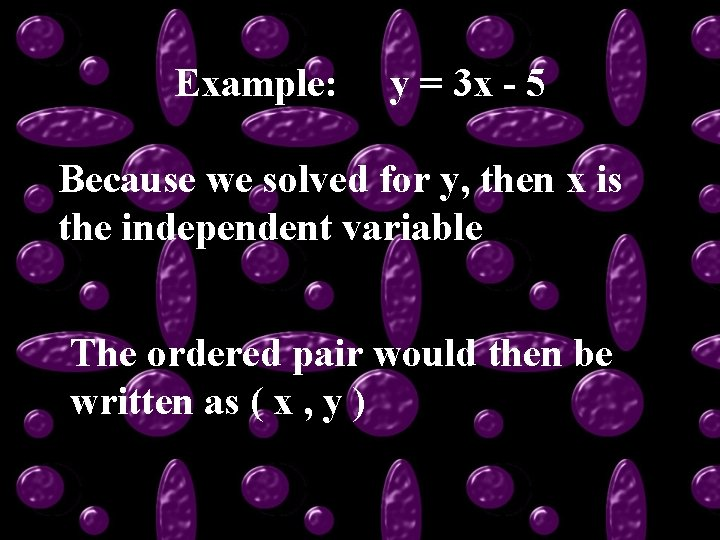Example: y = 3 x - 5 Because we solved for y, then x is the independent variable The ordered pair would then be written as ( x , y )If we do not know which variable is independent, then we choose one. No matter which one is independent, we will still produce similar ordered pairs ( r , s ) is similar to ( s , r ) Not congruent/equal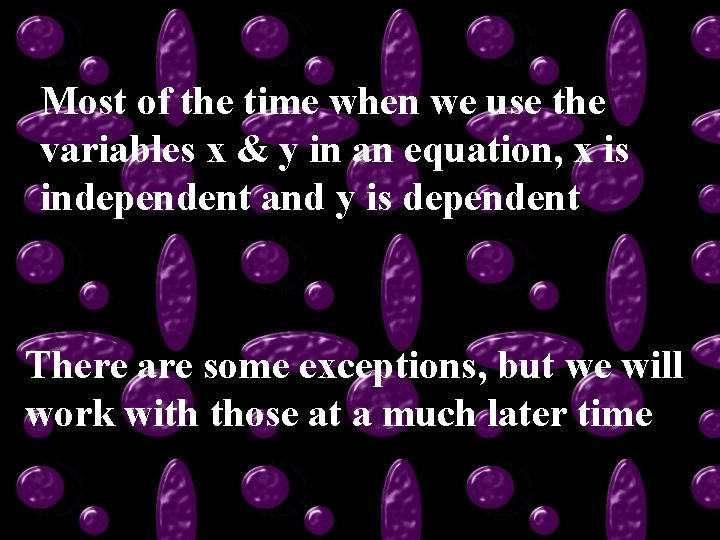Most of the time when we use the variables x & y in an equation, x is independent and y is dependent There are some exceptions, but we will work with those at a much later time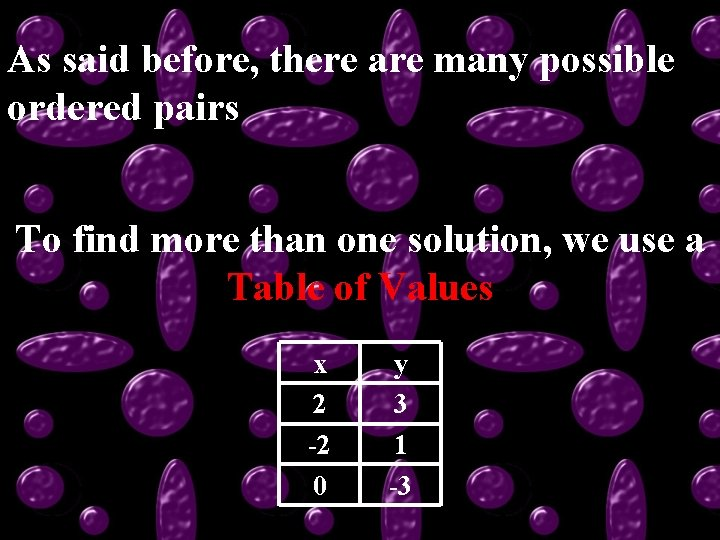As said before, there are many possible ordered pairs To find more than one solution, we use a Table of Values x 2 -2 0 y 3 1 -3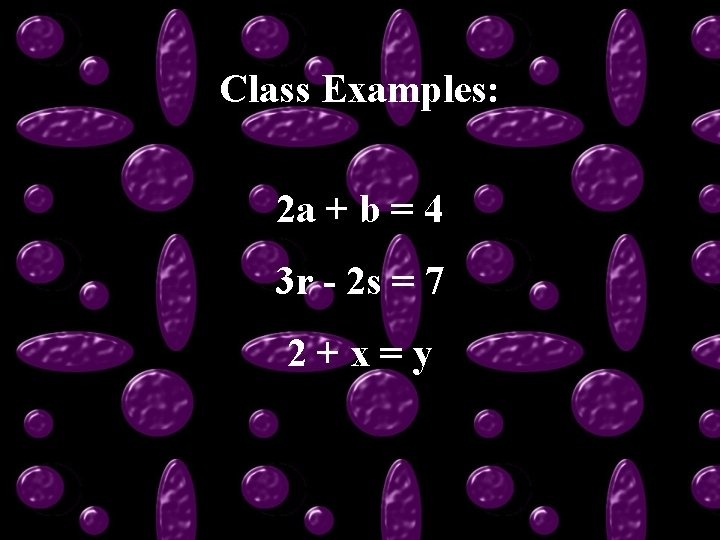Class Examples: 2 a + b = 4 3 r - 2 s = 7 2+x=yThis powerpoint was kindly donated to www. worldofteaching. com http: //www. worldofteaching. com is home to over a thousand powerpoints submitted by teachers. This is a completely free site and requires no registration. Please visit and I hope it will help in your teaching.